Tuesday, 4 Oct 2022

# What is the instantaneous rate of change ?

So in mathematics, physics, chemistry, economics, or in such other subjects, curves are a fundamental part. Therefore, what do curves mean? Well, whenever you have a collection of random data on the subject that you are working on and then plot them on the graph in the corresponding axes, you get a curve. However, you might get a straight line as well. But that is a rare case. This is because getting a straight line means your values have changed at a constant rate. However, that is quite impossible in real life. So, we have devised means to find the instantaneous rate of change. But the question is what exactly is the change that we are talking about.Therefore, let us consider that you are tracing the movements of a car. So, on the y-axis, you plot the distance that the car covers, and on the x-axis, you plot the time that it takes to cover the corresponding distance. This was the easy part. Now, plot the points. So, after this, join them. Here, two things can happen. You might get a straight line. This means the slope is constant or the instantaneous rate of change is 0. So, the car has been moving all the way with constant velocity. However, in the second case, you might get a curve. Moreover, this curve might be concave or convex depending on whether the car has increased or decreased speed. So, in this case, the slope is not constant. Therefore, the car has not moved with the same velocity throughout.

Read Also: What is the quotient and product rule ?

Now, you may need to find the change in velocity of the car at a particular point. So, for this, you have the instantaneous rate of change. We will see more of it in the next few sections.

## Instantaneous rate of change definition

As we all know, no matter how well we understand a topic, knowing the definitions in science is very important. So, before we delve deeper into the discussion of instantaneous rate of change, let us take a quick look at its definition. Therefore, the instantaneous rate of change is the change in the rate at a particular instant. Moreover, it is the same as the change in the derivative value at a specific point. So, what does this mean? This means that if you want to find the instantaneous rate of change of a car, you can simply find its derivative at that point. Moreover, we all already know that the slope of a line is basically its derivative. So that makes perfect sense.However, when you are drawing on a graph, and need to find the instantaneous rate of change at a particular point, the easiest way is to draw a tangent to it and find the slope. So, this is what we call a curve slope.

Now, we do not need this for just theoretical practice. There are various real-world applications of instantaneous rate of change. So, we have already seen one in the beginning. It might be the same when we find acceleration in a graph with time and velocity on the 2 axes. So, there are many such examples. Let us take a look at some of them but before that, we need to see the formula for how to find them. Knowing the formula is the most basic step because ultimately, whatever be the case, you need to use them in sums to get a proper answer.

## Instantaneous rate of change formula

Now you see, there is no particular formula to compute the instantaneous rate of change. So, the reason behind this is very simple. You are not exactly calculating any quantity but rather the rate of change at a point. So, you can use two ways to do this. Therefore, you might need to use calculus if you have a function at hand. So, at first, you differentiate the function. Now, you will also have the point at which you need to find the instantaneous rate of change. Therefore, substitute that point in the unknown value of the derivative. You will get the value of the rate of change. Let us take a look at a few examples where we need to find the value using calculus only. Then, we will go for the graphical method which is easier because you have the curve right before you.

### Instantaneous rate of change calculus.

Question 1: Find out the instantaneous rate of change of the function f(x) = 3x^2 + 12 at x = 4 ?

Answer: So, in this case, the point at which you need to find the instantaneous rate of change is x = 4. Therefore, let us differentiate the function at first.

f’(x) = 6x, the derivative of any constant is 0. So, we eliminate 12.

Now, we have to replace the value of x with 4.

So, f’(4) = 6 * 4 = 24.

Therefore, the instantaneous rate of change is 24.

Question 2: Find out the instantaneous rate of change of the function f(x) = 5x^3 – 4x^2 + 2x + 1 at x = 2?

Answer: So, we have the function f(x) = 5x^3 – 4x^2 + 2x + 1 and we have the point x = 2 where we need to find our final value.

Therefore, let us differentiate f(x) first.

f’(x) = 15x^2 – 8x + 2, the derivative of any constant is 0. So, we eliminate 1.

Now, we have to replace the value of x with 2.

So, f’(2) = 15 * (2^2) – 8 * 2 + 2 = 60 – 16 + 2 = 46.

Therefore, the instantaneous rate of change is 46.

Question 3: Find out the instantaneous rate of change of the function f(x) = sin^2x + cos^2x at x = 90 degrees.

Answer: So, here we have the function f(x) = sin^2x + cos^2x which is a little different from the previous ones because it is a trigonometric function. Moreover, we have to find the final value at 90 degrees.

So, let us differentiate f(x) first.

f’(x) = 2sinxcosx – 2cosxsinx, following the derivatives of trigonometric functions and the power rule.

Now, we replace x with 90 degrees.

So, f’(90) = 2sin90cos90 – 2cos90sin90 = 0 – 0 = 0.

## Note:

In the above example, the derivative comes to be 0. So, this means the instantaneous rate of change is also 0. Now, this makes sense. If we look at the trigonometric identity, sin^2x + cos^2x gives a constant value. Moreover, if you differentiate a constant value, you will always get a 0.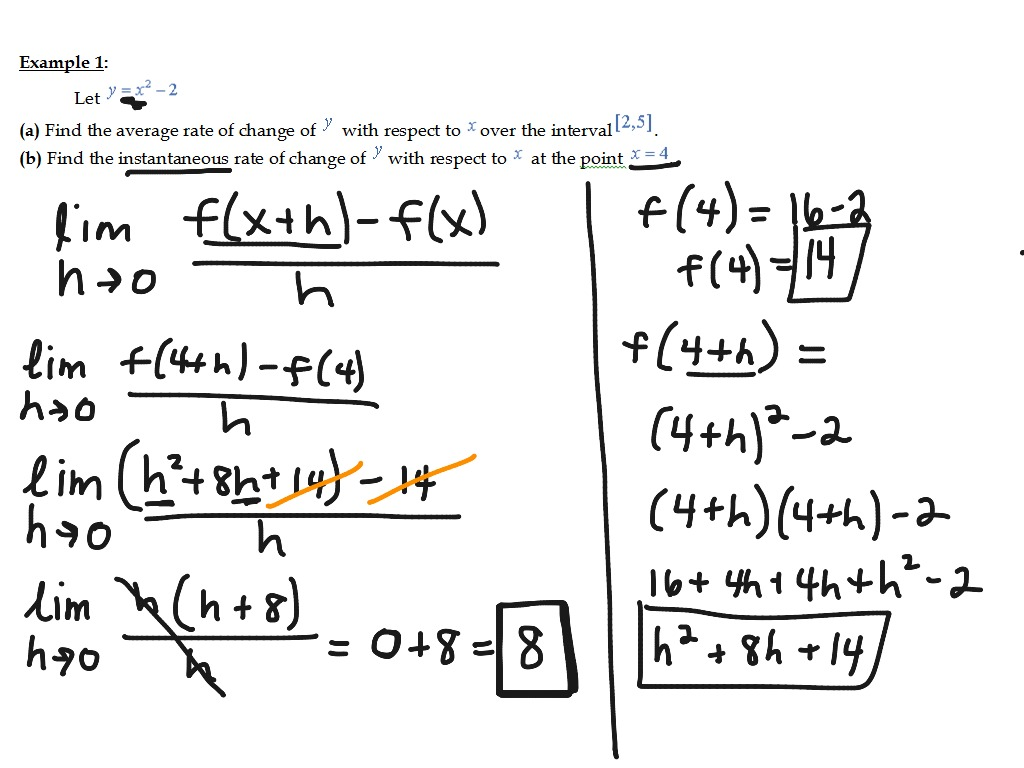## Instantaneous rate of change from graph.

Now, if you want to find the instantaneous rate of change from a graph, at first you have to draw a tangent to that point where you want the value. So, a tangent is a straight line that touches the curve perpendicularly at one point. Now, you have to find the slope of the tangent to get the final value at that point.

If the line was straight, you could have simply taken up two points on the line and found their difference to get the slope. However, that is not possible with curves because the rate is continuously changing. So, ultimately it boils down to calculus. You can also use the mean value theorem to calculate the rate of change over a particular interval. Moreover, you can also compare it with the average rate of change. The formula for finding the mean value using the mean value theorem is f’(c) = (f(b) – f(a)) / (b – a) where c = x.

Now, let us take a look at some real-life examples to understand its purpose or why exactly we need it in a better way.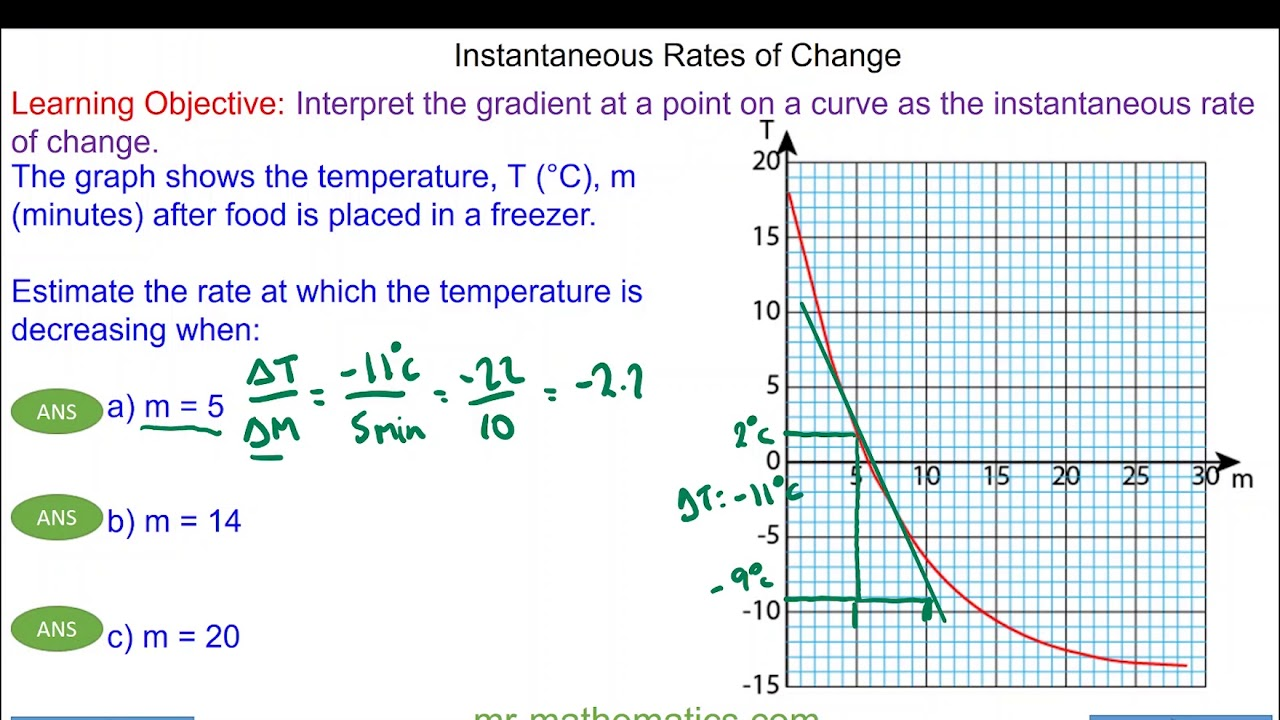## Instantaneous rate of change example

So, let us consider the acceleration of a car. A car is moving on a straight road in a particular direction but is constantly changing its speed. Once, it increases the speed and the other moment it decreases it. Therefore, the velocity is not changing at a constant rate. So, we do not have a fixed acceleration of the car. If we plot velocity on the y-axis and time taken on the x-axis and then try plotting the points, we will get a curve. However, this curve will follow no pattern whatsoever. This is a very real-life situation. Cars moving with constant acceleration or constant velocity over long distances only happen in ideal situations. So, such situations are what we start with when we are in school but when you are on the road, it is not that easy.

Therefore, you might need to find the change in acceleration at say, 5 seconds from the starting point. So, this is where the instantaneous rate of change finds application. You can find this value for every point on the curve and they will not be the same. They might be the same for small fragments of time because the car needs some time to accelerate, otherwise not.

Moreover, calculus is ideal for such a case. This is because we know we use calculus when the quantities are extremely small which is the case here.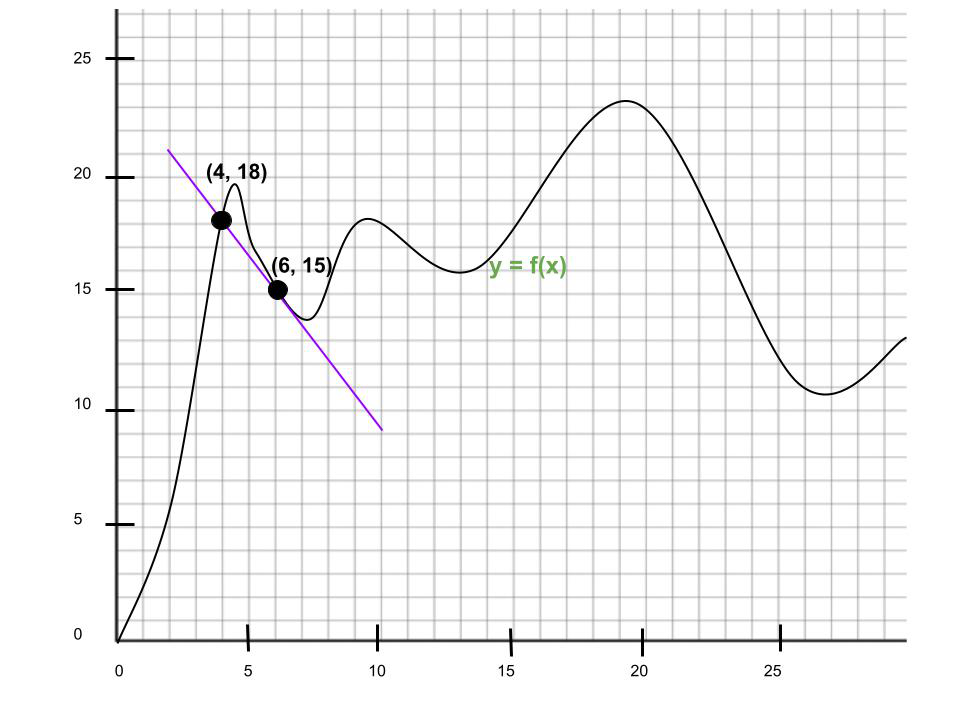## Instantaneous rate of change using limits

So, you can represent the instantaneous rate of change with the help of limits. Let us check how.

f’(a) = lim x->0 dy/ dx = lim x->0 [t (a + h) – (t(a))] / h.

So, here dy/dx is basically the ratio of the rate of change on the y-axis to that on the x-axis. Moreover, a is the concerned function here from which we have to find the final value.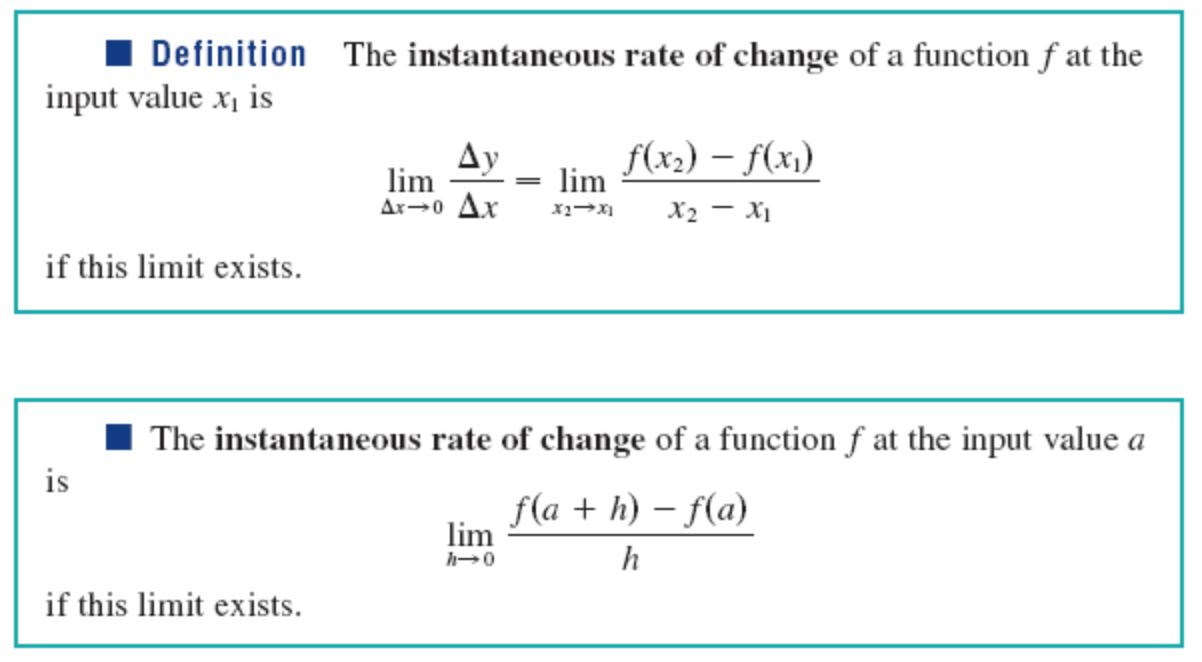## Instantaneous rate of change calculator

The benefit of everything being online these days helps us a lot. So, if you still have problems in understanding how to find out the instantaneous rate of change, you might use a calculator for better understanding. On the other hand, you might want to use one if you want to verify your own solution is correct. If not, you can also see where you went wrong. Therefore, let us see the steps we need to perform for using such a calculator. It is very simple and there are many options out there to suit you.

## Step 1 to use the instantaneous rate of change calculator

Go to the instantaneous rate of change calculator from Byju’s. Click on Byju’s and you will land directly on the calculator. Once you have reached the page, you will find a small blue box that looks like a dialog box. So, this is the instantaneous rate of change calculator.

## Step 2 to use the instantaneous rate of change calculator

Now, you see the calculator has two parts. So, in the first box enter the function or the equation of the curve. Therefore, come to the second line where there are two boxes. Here you have to specify the point of the variable that you have used in the equation, x for example.

Let us consider the function to be x^2 – x + 1 at x = 3. So, if you have completed the process, click the blue button underneath that reads “Find instantaneous rate of change.”

## Step 3 to use the instantaneous rate of change calculator

So, it will take a few seconds to compute the value and give you the final value. However, a new window will open. In the lower half of the window, you will see the solution. In this case, the answer is 5. However, you will not be able to see the steps directly.

## Step 4 to use the instantaneous rate of change calculator

If you want to see the solution step by step, click the option that provides so in the solution box. So, it will take a few seconds and you will end up in a different window to see the steps. This is helpful because without the steps you cannot understand the sum properly. Moreover, if you are using the calculator for verification and the answer does not match, you will know where you went wrong.

Now, for this case-

The function was f(x) = x^2 – x + 1.

So, let us find its derivative first.

Therefore, f’(x) = 2x – 1, the derivative of a constant is 0, so we eliminate 1.

Now, we substitute the value of x with 3.

So, f’(3) = 3 * 2 – 1 = 5.

Therefore, 5 is the final answer as we have already seen in the previous step.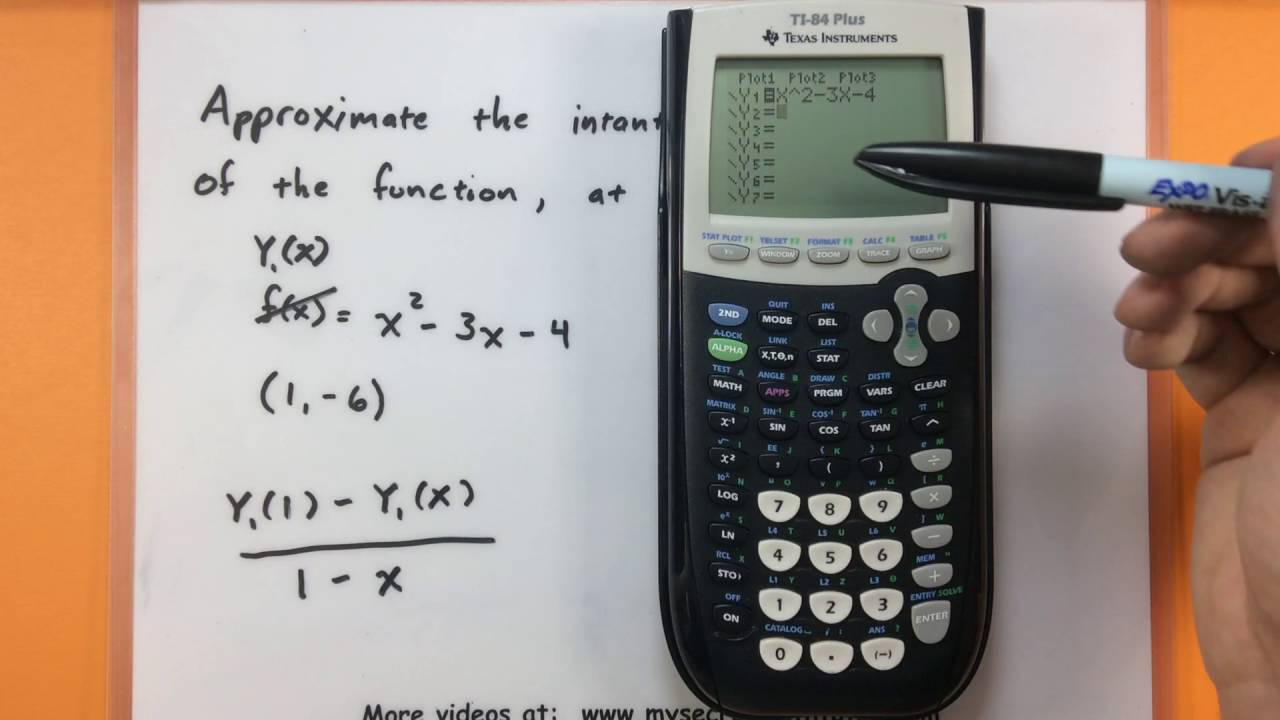## Instantaneous rate of change and average rate of change

There is a very basic difference between the two. So, for the average rate of change, you need to find the total change of one quantity with respect to another quantity. Let us take an example for easier understanding. Let us say you have a curve after plotting displacement against time. So, you do not have a constant velocity. However, you can find the average rate of change by simply dividing the total displacement by the total time taken. However, this is very different from the instantaneous rate of change where you need to consider very small quantities. So, a simple ratio will not work here to find the change at a particular point in time. Therefore, the change might be infinitesimally small. Moreover, it is constantly changing. Hence, we have to resort to calculus in this case.## Instantaneous rate of change FAQs

### Q. When can you not determine the instantaneous rate of change?

Ans. So, the best way to answer is when you cannot calculate the limit h->0 [f(x + h) – f(x)] / h or when it is not valid.

### Q. Why is instantaneous rate of change important?

Ans. So, this is because the instantaneous rate of change is analogous to a particular point. Therefore, it also explains why some points have an undefined rate of change.

### Q. What are the two ways to determine the instantaneous rate of change?

Ans. So, you can calculate it either via calculus to get the derivative of a function or from a graph by the slope of a tangent line to the curve. Moreover, we have already seen the process of finding both methods in detail. Scroll up and check the “calculus” and “from graph” sections for better understanding.

### Q. What is the difference between the average rate of change and instantaneous rate of change?

Ans. So, the average rate of change gives you the rate of change over a much longer period. Moreover, it calculates the slope of the secant line using the algebraic formula of finding a slope. On the other hand, for the instantaneous one, you are calculating the change at a particular point. So, the change is infinitesimally small. Moreover, you find this by calculating the slope of the tangent line.

### Q. Is instantaneous change possible?

Ans. If your question means whether instantaneous change is possible at a single point, it might be a no. So, in real life for instantaneous change in position, you would need infinite velocity. Moreover, for instantaneous change in velocity, you would need infinite acceleration. However, gaining infinite velocity or infinite acceleration is quite impossible in reality.

### Q. Is instantaneous rate positive or negative?

Ans. So, the instantaneous rate of change can be either positive or negative. If the change denotes an increase, it will be positive. However, if it denotes a decrease, it will be negative. Moreover, it can also be zero if there is no change.